# Activation Functions for Output Layer in Neural Networks

Designing a neural network architecture involves selecting the appropriate activation functions for hidden and output layers. The correct activation function choice can dramatically change a neural network's performance.

Our previous blog discussed the possible options for hidden layer activation functions. Here, we will focus on understanding the possible ways to select the appropriate activation function for the output layer.

### What is the Output layer?

The output layer is the last layer in the neural networks. It receives input from the previous hidden layer (if present) in the architecture or directly from the Input layer and transforms it into the desired output. The desired output can be a single floating number in case of regression problems or a vector of probability values in classification problems.

Neural Networks are supervised learning algorithms where we pass input vectors along with their corresponding output values, known as labels. Let's call it Y_actual. In a neural network architecture, most "learnings" happen in the hidden layers where the input vector gets significantly transformed (after passing through multiple hidden layers).

The output of these hidden layers is passed to the output layer, where networks further transform this input into a final form in which labels are present. The final output from the Output layer is the predictions the entire neural network makes. Let's call it Y_predicted.

``````H_last = Last Hidden layer output
Wo = Weight of Output layer
Bo = Bias of Output layer
act_fn = Activation function

Y_predicted = act_fn(Wo * H_last + Bo)``````

Now, the values of Ypredicted and Yactual will be used to calculate the cost function. For example, if we are solving a regression problem and the cost function we chose is MSE, then:

``````## In Machine learning, Cost function is represented with J(θ)

J(θ) = Σ (Y_actual - Y_predicted)^2``````

Now the operation of optimization algorithms like gradient descent starts, where they find the derivative of this cost function to update the parameters of the whole network.

The choice of the appropriate activation function plays a vital role in forming the final form of predictions. Here, this function's choice depends on the task we perform using our neural networks.

Neural Networks are supervised algorithms. So, based on the nature of the problem statement, we can define the task into 2 major categories: Classification and Regression. If one wants to read the detailed blog on these categories, please find it in the Classification and Regression in machine learning blog.

Let's start with the regression problem and see which activation functions will be the perfect candidate.

### Choices of Activation Function for Regression Problems

In the Regression problem, machine learning models predict the continuous mapping function. Here we have one floating point value corresponding to every input value. For example, predicting weather temperature with a floating value of 24.5 °c.

As stated, most of the learning happens in the hidden layers, and the role of the output layer is to transform the output vector of the last hidden layer into:

• One single floating value if there is one input sample fed to the Input layer or
• A vector of floating values if the model is fed with a batch of inputs. Usually, a batch contains 16, 32 or 64 input samples, and we have corresponding 16, 32 or 64 outputs, respectively.

Now, if the goal is not to learn much here, we can think of a method that will save the computational complexity of the overall network. This makes the Linear activation function a great choice, so let's learn it in detail.

#### 1. Linear Activation Function

The linear activation function is one of the perfect candidates that can be used directly in the output layer if we are solving regression problems. It is also known as the 'identity' function and treated as 'no-activation'. Let's see its mathematical formula and graph, which will justify the reason for that.

``linear(x) = x, where x ∈ (-∞, + ∞)``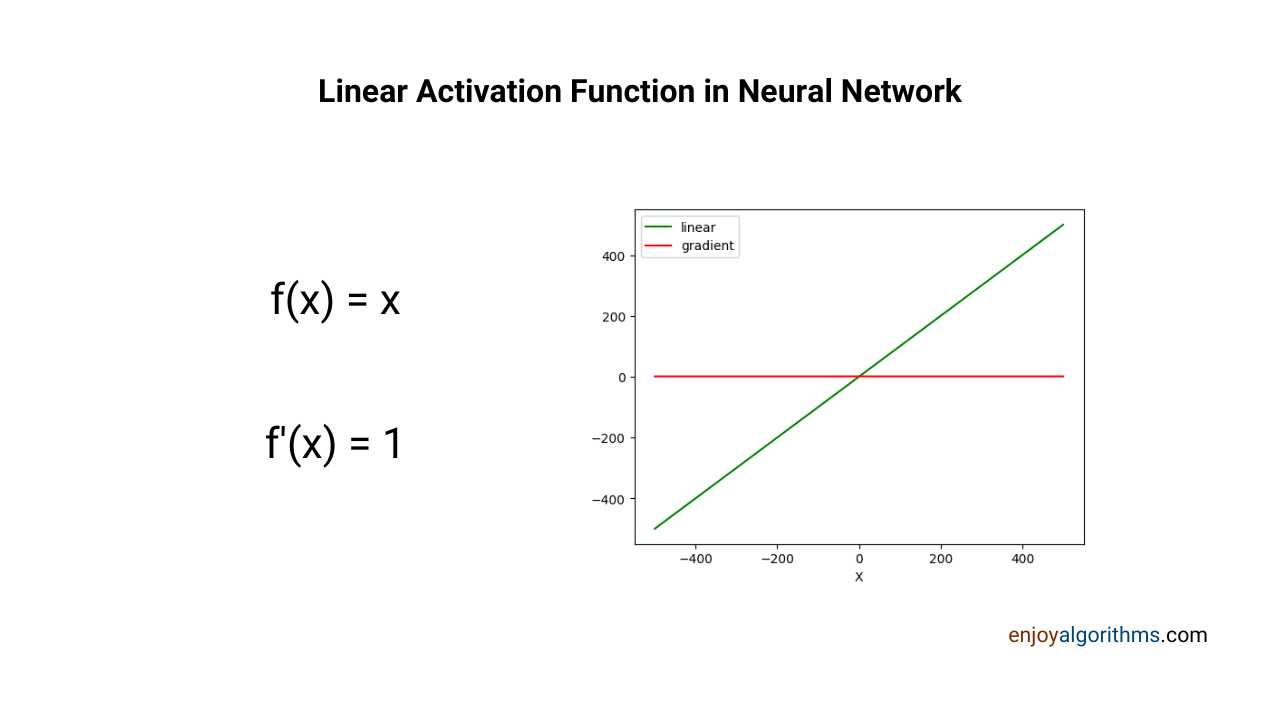By looking at the mathematical formula and graphical representation, we can easily infer that the function and its gradient both are computationally very cheap and helps fasten the process of Neural Networks. Let's see the Python implementation of the Linear activation function from scratch:

``````from matplotlib import pyplot as plt
import numpy as np

def linear(x):
return x

return 1

x = [t for t in np.arange(-500.0, 500.0, 0.01)]
y = [sigmoid(i) for i in x]

plt.figure('Sigmoid Activation Function')

plt.plot(x, y, 'g', label='sigmoid')
plt.legend(loc='best')
plt.xlabel('X')

plt.show()``````

Let's also learn the method from the Keras library, which we will be using while building ANN applications:

``````import tensorflow as tf
tf.keras.activations.linear(x)``````

There can be other options like ReLU, but Linear will still be the preferred choice as it will be computationally better.

#### Choices of Activation Function for Classification Problems

In a classification problem, we categorize incoming inputs into various classes. For example, a neural network can take an input image and provide the output of whether a cat is present in that image or not. There can be three types of classification problems:

• Binary classification problem: Here, the number of classes is two, and both classes form a complementary set. A complementary set is something which completes the other. For example, there can be only two scenarios: 1. The Cat is present in the image, 2. The cat is not present in the image. These two classes, present and not present, are complementary. Some popular industrial applications of binary classification are 1. Email Spam and Non-spam Classification 2. Cancer Classification.
• Multi-class classification problem: Here, the number of classes is more than two and machine-learning models give predictions as confidence values for each class. For example, in the image below, a classifier model gives predictions as confidence scores. For the image of an apple, the ML model says that it is 10% confident that the input is a banana, 90% sure that the input is an apple, and 50% sure that it is a cherry. The model outputs the highest confidence class as the final prediction.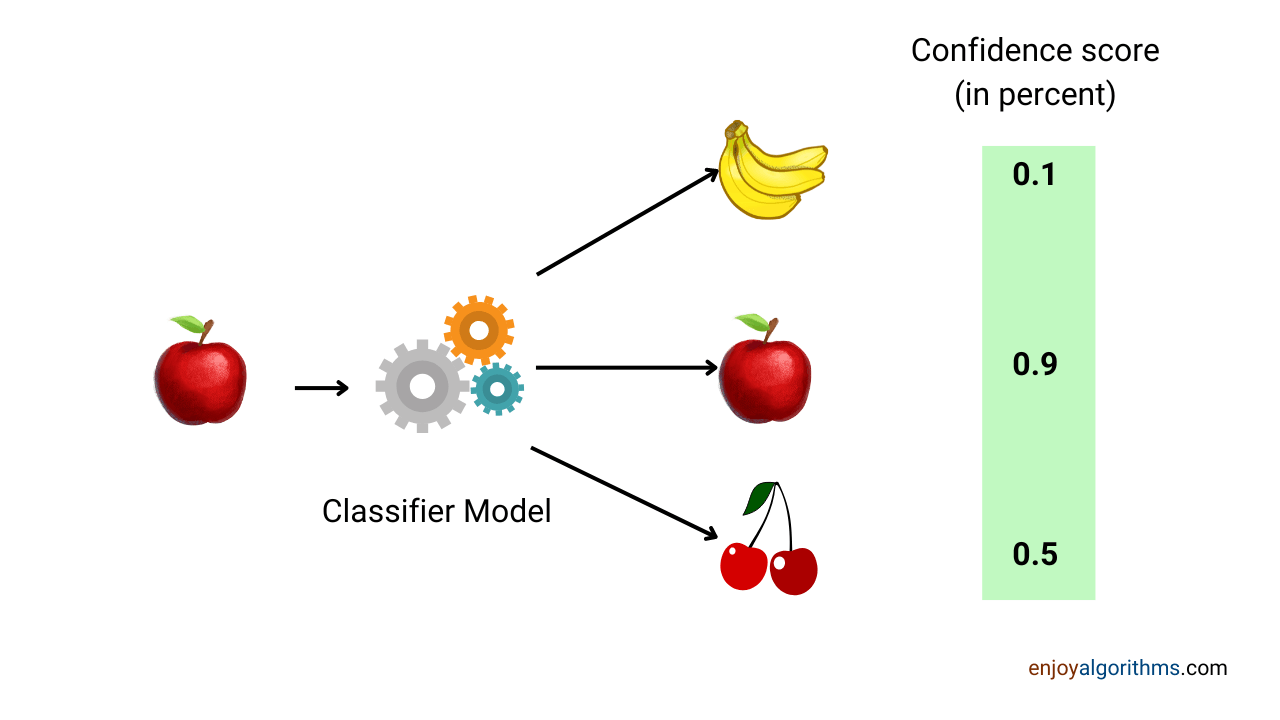The ML model will provide only one final class as the output in case of multi-class classification problems. Some popular industrial applications for this category are 1. Uber Surge price prediction 2. Sentiment Analysis

• Multi-label classification problem: Here, the number of classes is more than 2, but the same input can give predictions about multiple classes. For example, one image can contain multiple labels at the same time. The ML models treat every label as a binary classification problem with assumed types of label present or not present. If the confidence for this prediction is above the given threshold, the model predicts that label. For example, if the ML model predicts a 70% confidence score for all the labels and there is a threshold of 60% (means if the prediction is above 60% for any label, the model will include that label in the final prediction), then it will predict all the labels.

The difference between multi-class classification and multi-label classification is shown in the image below.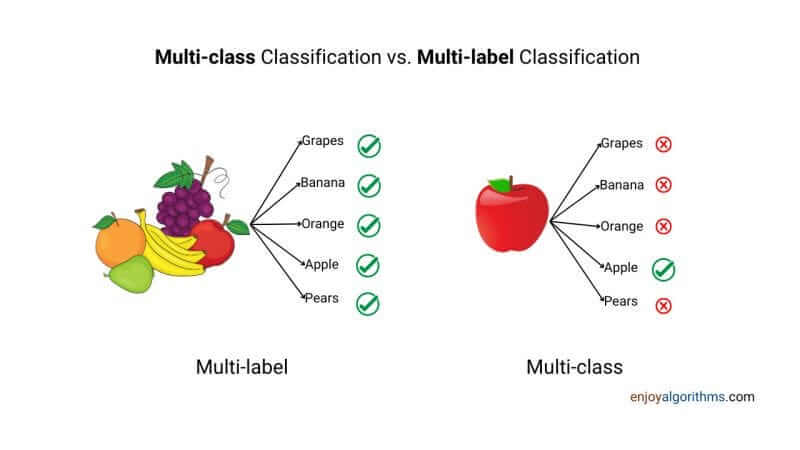Some popular industrial applications for this category are 1. Optical character recognition.

Now, we need to choose the activation function carefully for each of these three categories of classification problems, as this can change the prediction. For classification problems, we generally prefer either of the two activation functions:

1. Sigmoid
2. Softmax

#### Sigmoid Activation Function

We have discussed the Sigmoid activation function in greater detail while discussing the activation functions for hidden layers, and one can find the details here.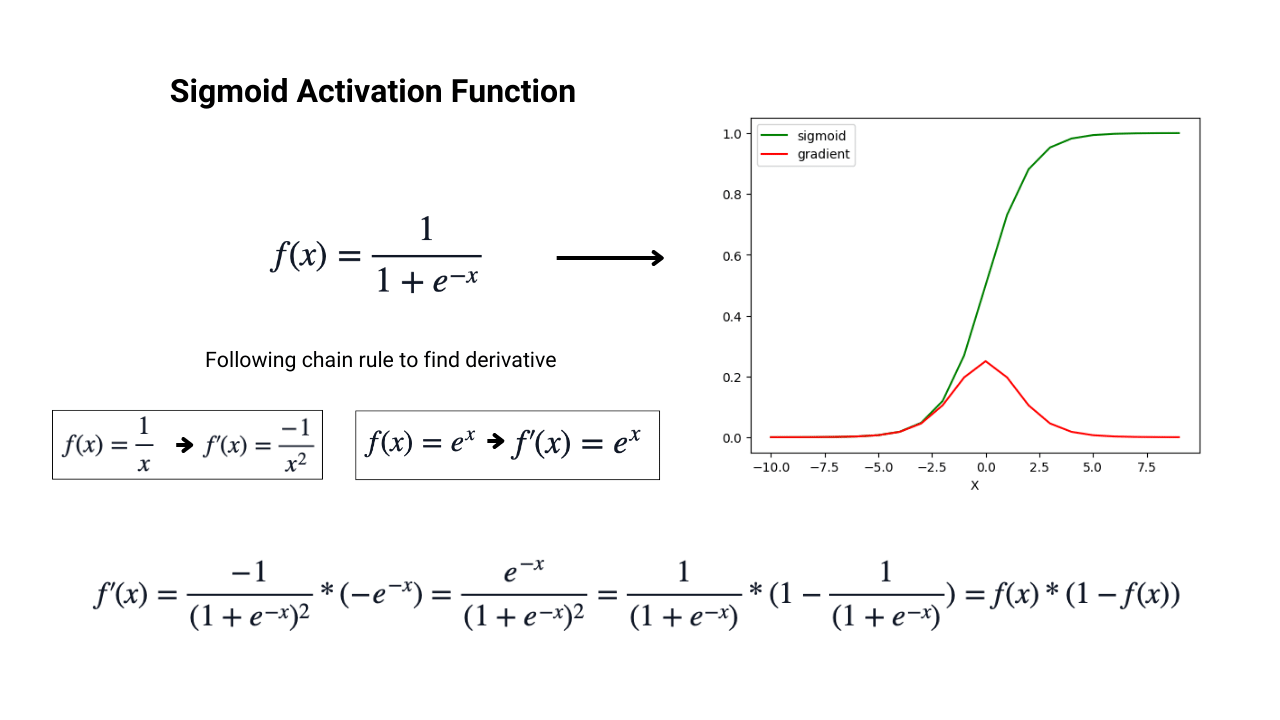``````import tensorflow as tf

x = [t for t in np.arange(-500.0, 500.0, 0.01)]
out = tf.keras.activations.sigmoid(x)``````

The output of the sigmoid activation function is confined in the range of (0,1), which makes it a perfect candidate for the output layers to predict probabilities of various classes.

This probability can be treated as the confidence score, and if the confidence in prediction is beyond the threshold, the model predicts that label. For example, if the model says that the probability of cat presence in the image is more than 60%, it will predict that the cat is present as the final output.

#### Softmax Activation Function

Softmax is the most preferred activation function for the output layer when we solve the multi-class classification problem using machine learning. It produces an output vector corresponding to the probability values for classes in multi-class classification problems. The sum of all probabilities corresponding to every class is 1.0.

This activation function takes the input vector with the shape of N x M, where N is the number of samples and M is the number of labels present in a multi-class problem. As a result, it produces the output of the same shape, N x M, but this time, each sample's 'M' values will be probabilities for M classes. The mathematical formula for softmax is: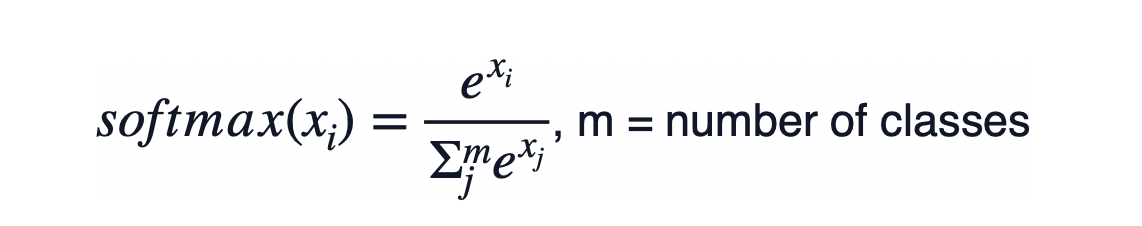In simple words, softmax converts the 'm' numerical values into 'm' probabilities, with the sum of all these m probabilities being 1. We can not plot this activation function or its derivative. So let's see the implementation in Python.

``````import numpy as np

def softmax(x):
sum_total = 0
for i in x:
sum_total += np.exp(i)

y = [np.exp(i)/sum_total for i in x]

return y

x = [1, 3, 5, 7, 9, 0]
y = softmax(x)

print(y)

# [0.00029004491601598327, 0.0021431581556517294, 0.015835915840991373, 0.1170124705270298, 0.8646117089986927, 0.00010670156161857785]

print(sum(y))

# 1.0``````

We shall notice in the code above that the sum of softmax output produces 1. Let's see the implementation using the Keras library as well.

``````import tensorflow as tf

x = tf.random.normal(shape=(32, 10))
y = tf.keras.activations.softmax(x)

one_sample = tf.reduce_sum(y[0, :])
print(one_sample)

# tf.Tensor(1.0000001, shape=(), dtype=float32)``````

We need to note that the softmax activation function from the Keras library only accepts tensors with 2D shapes as input, and when we print the sum of probabilities for each sample, it gives 1. The total number of classes in the example code is 10.

Now the main gist of this entire blog is, "How to decide the activation function for the output layer?" so let's see.

### How to Decide the Activation Function for the Output Layer?

We have learnt that for regression problems, the linear layer is the best choice as it is computationally efficient. For classification problems, if the problem is:

#### Binary classification:

Sigmoid is preferred for solving binary classification problems over softmax activation function, and the reason for that is, Sigmoid is computationally cheaper than Softmax.

If we pick Sigmoid as the activation function for the binary classification problem, the output layer will have 1 node. But in the case of the softmax activation function, it needs 2 output nodes [probability of true, probability of false]. The presence of 2 nodes will involve higher dimensional matrix multiplication, and hence Sigmoid will be computationally efficient.

#### Multi-class classification:

Softmax is the best choice for solving multi-class classification problems. The total number of nodes that will be present in the output layer will be equal to the total number of classes present, meaning one node per class. The softmax function will output the vector with probabilities; as a final prediction, it will predict the class, whichever has the highest probability.

We can also use Sigmoid to solve the multi-class problem, but here we need to divide each class into two categories and handle each class as a binary classification problem.

#### Multi-label classification:

Sigmoid is the only choice to solve the multi-label classification problem. Here model predicts all the labels for which the probability value is greater than a certain threshold. For example, if the model says that there is a 60% probability of cat presence and a 70% probability of dog presence, then the model will predict both cat and dog for that image.

Here the total number of nodes will equal the number of classes present, meaning one node per class.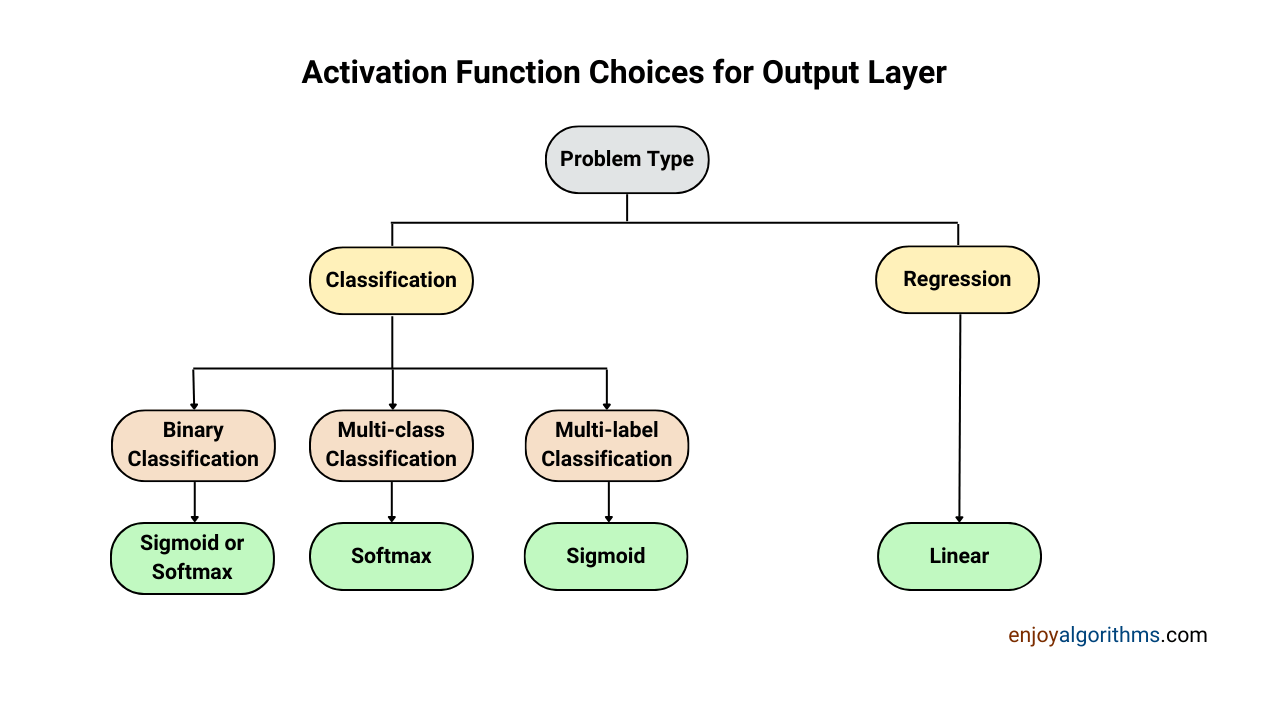### Conclusion

While designing the neural networks, we choose the activation function for the output layer, which highly depends on the nature of the problem statement we are solving. For the regression problem, we have a linear activation function. For the classification problem, we choose between Sigmoid and Softmax based on the classification problem we are solving. We hope you find the article informative and enjoyable.

☆ 16-Week Live DSA Course
☆ 10-Week Live DSA Course